GFG App
Open AppBrowser
Continue

# Class 9 NCERT Solutions – Chapter 13 Surface Areas And Volumes – Exercise 13.1

### (i) The area of the sheet required for making the box.

Solution:

Given,

Length of box (l) = 1.5 m

Breadth of box (b) = 1.25 m

Height of box (h) = 65 cm = 0.65 m and box is open at top,

Therefore, area of sheet required = lb + 2lh + 2bh

= (1.5 * 1.25 + 2 * 1.5 * 0.65 + 2 * 1.25 * 0.65)m2

= (1.875 + 1.95 + 1.625)m2

= 5.45 m2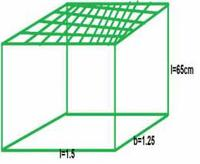### (ii) The cost of thesheet for it, if a sheet measuring 1m2 costs 20 rupees.

Solution:

The area of the box is 5.45 m2

Given, 1 m2 costs 20 rupees

Therefore, cost of 5.45 m2 box is = (5.45 * 20) rupees

= 109 rupees

### Question 2: The length, breadth and height of a room are 5 m, 4 m and 3 m respectively. Find the cost of whitewashing the walls of the room and the ceiling at the rate of Rs. 7.50 per m2.

Solution:

Given,

Length of room (l) = 5 m

Breadth of room (b) = 4 m

Height of room (h) = 3 m

Area of walls + Area of ceiling = lb + 2lh + 2bh

= (5 * 4 + 2 * 5 * 3 + 2 * 4 * 3)m2

= (20 + 30 + 24)m2

= 74 m2

Cost of white wash per 1m2 = 7.50 rupees

Cost of white wash per 74m2 = (74 * 7.50) rupees

= 555 rupees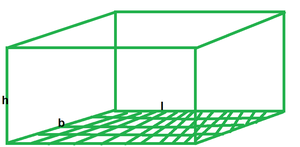### [Hint: Area of the four walls = Lateral surface area.]

Solution:

Area of 4 walls = 2lh + 2bh

= 2 (l + b) h

Perimeter of the floor of the hall = 2 (l+ b) = 250 m (given)

Therefore, area of 4 walls = 250h m2

Cost of painting per 1m2 = 10 rupees

Cost of painting per 250h m2 = (250h * 10) rupees

= 2500h rupees

Given, cost of painting for 4 walls is 15000 rupees

Therefore, 2500h = 15000

h = 15000 / 2500

h = 6

Height of the hall is 6m.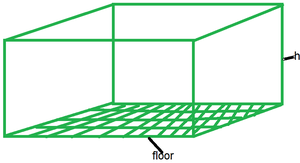### Question 4: The paint in a certain container is sufficient to paint an area equal to 9.375 m2. How many bricks of dimensions 22.5 cm × 10 cm × 7.5 cm can be painted out of this container?

Solution:

Total surface area of each brick = 2 (lb + bh + lh)

= 2 (22.5 * 10 + 10 * 7.5 + 7.5 * 22.5) cm2

= 2 (225 + 75 + 168.75) cm2

= 937.5 cm2

= 0.09375 m2

Given, the paint is sufficient to paint 9.375 m2

Therefore, number of bricks that can be painted = (9.375 / 0.09375)

= 100.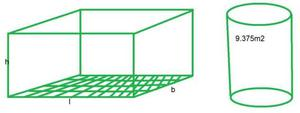### (i) Which box has the greater lateral surface area and by how much?

Solution:

Given, edge of a cube = 10 cm

Lateral surface area of a cube = 4 * (edge)2

= 4 * 102 cm2

= 400 cm2

Given, Length of cuboid (l) = 12.5 cm

Breadth of cuboid (b) = 10 cm

Height of cuboid (h) = 8 cm

Lateral surface area of a cuboid = 2 (lh + bh)

= 2 (12.5 * 8 + 10 * 8) cm2

= 2 (100 + 80) cm2

= 360 cm2

Therefore, lateral surface area of cubical box is greater than cuboidal box by (400 cm2 – 360 cm2) which is 40 cm2.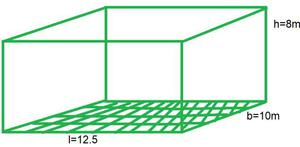### (ii) Which box has the smaller total surface area and by how much?

Solution:

Total surface area of a cube = 6 * (edge)2

= 6 * 102 cm2

= 600 cm2

Total surface area of cuboid = 2 (lb + bh + lh)

= 2 (12.5 * 10 + 10 * 8 + 12.5 * 8) cm2

= 2 (125 + 80 + 100) cm2

= 610 cm2

Therefore, total surface area of cuboidal box is greater than cubical box by (610 cm2 – 600 cm2) which is 10 cm2.

### (i) What is the area of the glass?

Solution:

Length of greenhouse (l) = 30 cm

Breadth of greenhouse (b) = 25 cm

Height of greenhouse (h) = 25 cm

Area of glass = total surface area of greenhouse

= 2 (lb + bh + lh)

= 2 (30 * 25 + 25 * 25 + 30 * 25)

= 2 (750 + 625 + 750)

= 4250 cm2

Therefore, area of glass is 4250 cm2.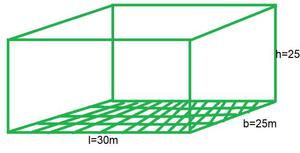### (ii) How much of tape is needed for all the 12 edges?

Solution:

Length of tape needed = perimeter of the greenhouse

= 4 (l + b + h)

= 4 (30 + 25 + 25) cm

= 320 cm.

Therefore, length of tape needed is 320 cm.

### Question 7: Shanti Sweets Stall was placing an order for making cardboard boxes for packing their sweets. Two sizes of boxes were required. The bigger of dimensions 25 cm × 20 cm × 5 cm and the smaller of dimensions 15 cm × 12 cm × 5 cm. For all the overlaps, 5% of the total surface area is required extra. If the cost of the cardboard is Rs. 4 for 1000 cm2, find the cost of cardboard required for supplying 250 boxes of each kind.

Solution:

Total surface of area of bigger cardboard box = 2 (lb + bh + lh)

= 2 (25 * 20 + 20 * 5 + 25 * 5) cm2

= 2 (500 + 100 + 125) cm2

= 1450 cm2

Total surface area of bigger cardboard box including overlaps = (1450 + 0.05 * 1450) cm2

= 1522.5 cm2

Area of 250 bigger boxes = (1522.5 * 250) cm2

= 380625 cm

Total surface area of smaller cardboard box = 2 (lb + bh + lh)

= 2 (15 * 12 + 12 * 5 + 5 * 15) cm2

= 2 (180 + 60 + 75) cm2

= 630 cm2

Total surface area of smaller cardboard box including overlaps = (630 + 0.05 * 630) cm2

= 661.5 cm2

Area of 250 smaller boxes = (661.5 * 250) cm2

=165375 cm2

Total cardboard sheet required = (380625 + 165375) cm2

= 546000 cm2

Given cost of 1000 cm2 Cardboard sheet = Rs. 4

Therefore, cost of 546000 cm2 Cardboard sheet = (546000 * 4) / 1000 rupees

= 2184 rupees.

The cost of cardboard required for supplying 250 boxes of each kind is 2184 rupees.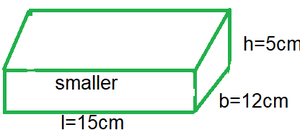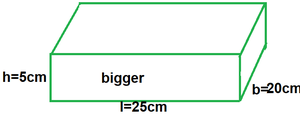### Question 8: Parveen wanted to make a temporary shelter for her car, by making a box-like structure with tarpaulin that covers all the four sides and the top of the car (with the front face as a flap which can be rolled up). Assuming that the stitching margins are very small, and therefore negligible, how much tarpaulin would be required to make the shelter of height 2.5 m, with base dimensions 4 m × 3 m?

Solution:

Length of the shelter (l) = 4 m

Breadth of the shelter (b) = 3 m

Height of the shelter (h) = 2.5 m

Area of tarpaulin required = lb + 2lh + 2bh

= (4 * 3 + 2 * 4 * 2.5 + 2 * 3 * 2.5) m2

= (12 + 20 + 15) m2

= 47 m2

My Personal Notes arrow_drop_up